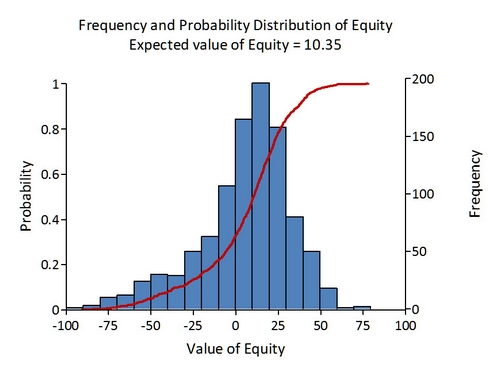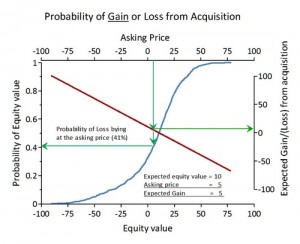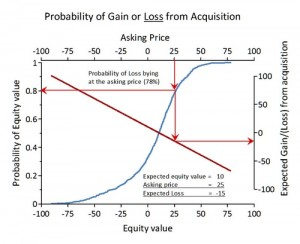# The Probability of Gain and Loss

Every item written into a firm’s profit and loss account and its balance sheet is a stochastic variable with a probability distribution derived from probability distributions for each factor of production. Using this approach we are able to derive a probability distribution for any measure used in valuing companies and in evaluating strategic investment decisions. Indeed, using this evaluation approach we are able to calculate expected gain, loss and their probability when investing in a company where the capitalized value (price) is known.

The Probability Distribution for the Value of Equity

The simulation creates frequency and cumulative probability distributions as shown in the figure below.We can use the information contained in the figure to calculate the risk of investing in the company for different levels of the company’s market capitalization. The expected value of the company is 10.35 read from the intersection between probability curve and a line drawn from the 50% probability point on the left Y-axis.

The Probability Distribution for Gain and Loss

The shape of the probability curve provides concise information concerning uncertainty in calculating expected values of equity. Uncertainty isreduced the steeper the probability curve, whereas the flatter the curve so uncertainty is more evident. The figures below depicts the value of this type of information enabling calculation of expected gains or losses from investments in a company for differing levels of market capitalization.

We have calculated expected Gain or Loss as the difference between expected values of equity and the market capitalization; the ‘S’ curve in the graph shows this. The X-axis gives different levels of market capitalization; the right Y-axis gives the expected gain (loss) and the left y-axis the probability. Drawing a line from the 50% probability point to the probability curve and further to the right Y-axis point to the position where the expected gain (loss) is zero. At this point there is a 50/50 chance of realising or loosing money through investing in the company capitalized at 10.35, which is exactly the expected value of the company’s equity.

To the left of this point is the investment area. The green lines indicate a situation where the company is capitalized at 5.00 indicating an expected gain of 5.35 or more with a probability of 59% (100%-41%).The figure to the right describes a situation where a company is capitalized above the expected value.

To the right is the speculative area where an industrial investor, with perhaps synergistic possibilities, could reasonably argue a valid case when paying a price higher than expected value. The red line in the figure indicates a situation where the company is capitalized at 25.00 – giving a loss of 14.65 or more with 78% probability.

To a financial investor it is obviously the left part – the investment area – that is of interest. It is this area that expected gain is higher than expected loss.